# Zenith and Azimuth

Stars may be identified by their position in the celestial dome. Think of declination and right ascension as extension of latitude and longitude and pointing to a star from earth is the same as pointing to a different location on earth except the line of sight is extended into the sky.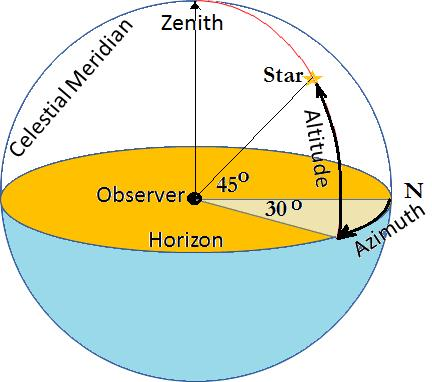Fig 1.22. Local coordinates system.
An observer standing at a known latitude and longitude looking straight ahead, can use the Compass north as a starting point. Looking straight ahead, the horizon is defined as an imaginary circle all around, while zenith is the point straight overhead. The altitude is the angle directly beneath the star on the horizon plane and the azimuth is the angle from geographic north to the point directly beneath star.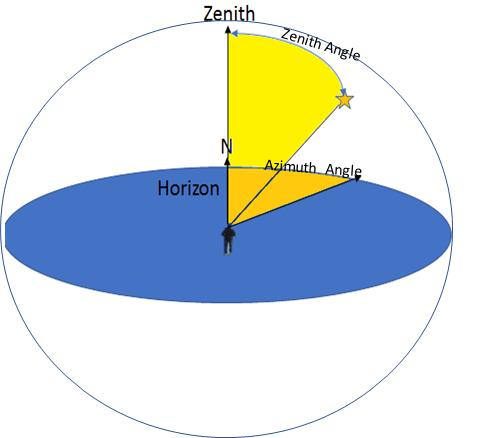Fig 1.23. Observing star from local coordinate system
It is also helpful to use the angle between zenith and the star called zenith angle as shown in the Fig.
To locate an object in the sky at a specific time, the zenith and azimuth angle with reference to local latitude and longitude must be calculated.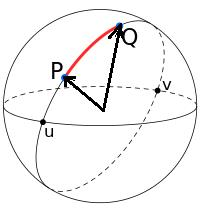Fig 1.24. Zenith angle
Suppose, P is the local reference point with known latitude and longitude and Q is the star declination and right ascension with respect to P. The angle between P and Q is the zenith angle as indicated with red color in the Fig.
Extend a line from the center to absolute North (point R) as shown in the Fig. Join P to R and Q to R.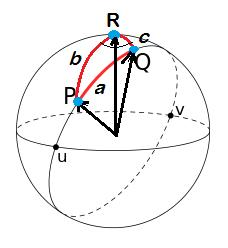Fig 1.25. Extending the arch from observer’s point P to north R and star’s location Q to north R.

Using the spherical law of cosines (for a spherical triangle with surface angles P, Q, R opposite sides corresponding to central angles a, b, c)

Zenith angle a = cos-1(cos b cos c + sin b sin c cos (PRQ))

The azimuth angle (RPQ) may be calculated Using the Dot Product Rule (the angle between the two Vectors PR and PQ).
PR ● PQ = PR*PQcosRPQ
azimuth angle ∠PRQ=cos-1PR●PQPR*|PQ|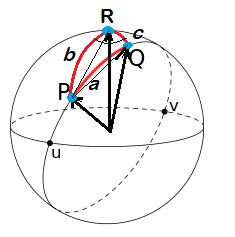Fig 1.26. Vector PR and PQ.

Visit http://starcoordinates.sahraid.com/ for converting celestial coordinates of right ascension and declination into horizon coordinates of zenith and azimuth angle.

Note

Notes

# 3D-Compass

You can make a 3-Dimensional compass with a paper cutout as shown in the Fig. and fold it to make a pointing device to point to an object in the sky.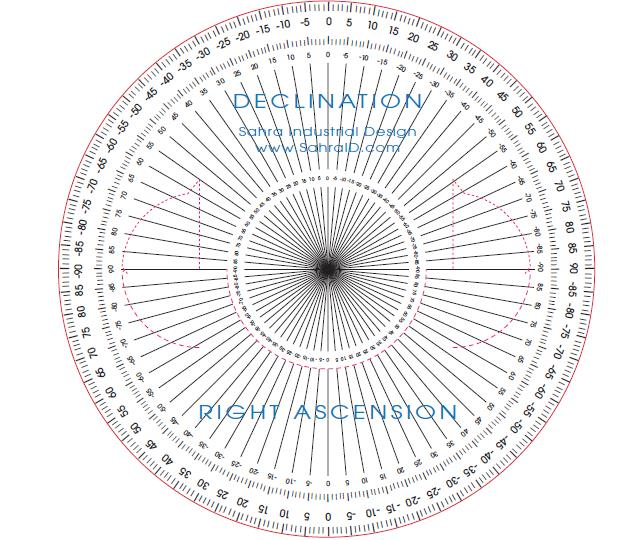Fig 1.27a. 3D Compass cutout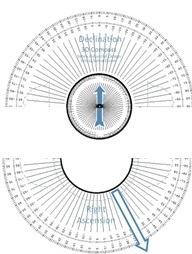Fig 1.27b. The declination half and the right ascension half

One-half is the horizontal declination plane and the other half is the vertical Right-Ascension plane. A compass is placed on the edge of the horizontal plane and a pointer on the vertical plane.
Fold the vertical half along the edge over the horizontal plane as shown in the Fig.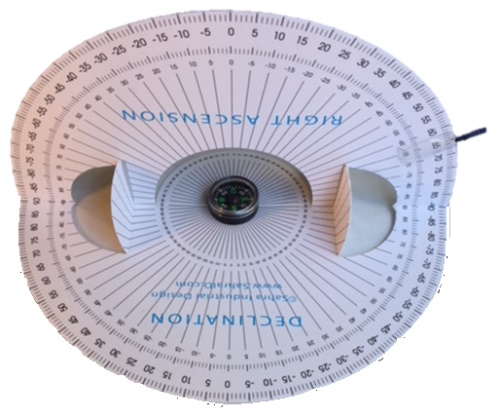Fig 1.28a. Fold 3D Compass cutout along declination half and right ascension half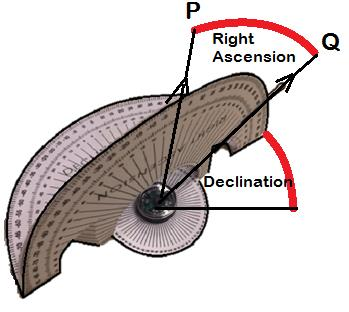Fig 1.28b. Position the declination plane horizontally, align 0o with compass north, move right ascension pointer to the zenith angle

Hold the 3D-Compass with compass north aligned with 0o declination. Position the right ascension pointer to the star’s zenith angle as shown in Fig. Rotate the compass horizontally till compass north points to the azimuth angle as shown in Fig. The right ascension pointer will point towards the star.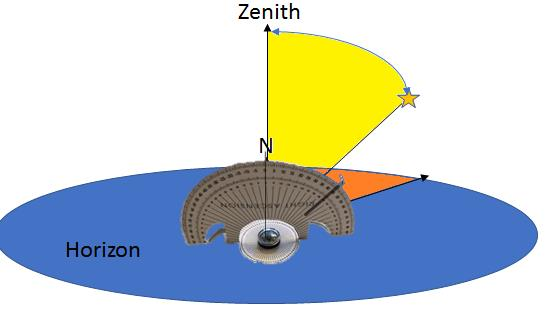Fig 1.29a. 3D Compass position right ascension pointer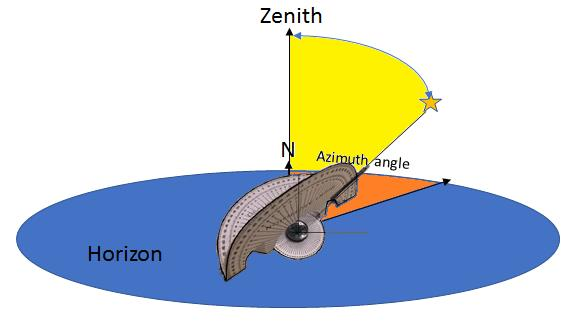Fig 1.29b. 3D Compass rotating to azimuth angle

Table 2. Star Coordinates and 3D Compass Arc angles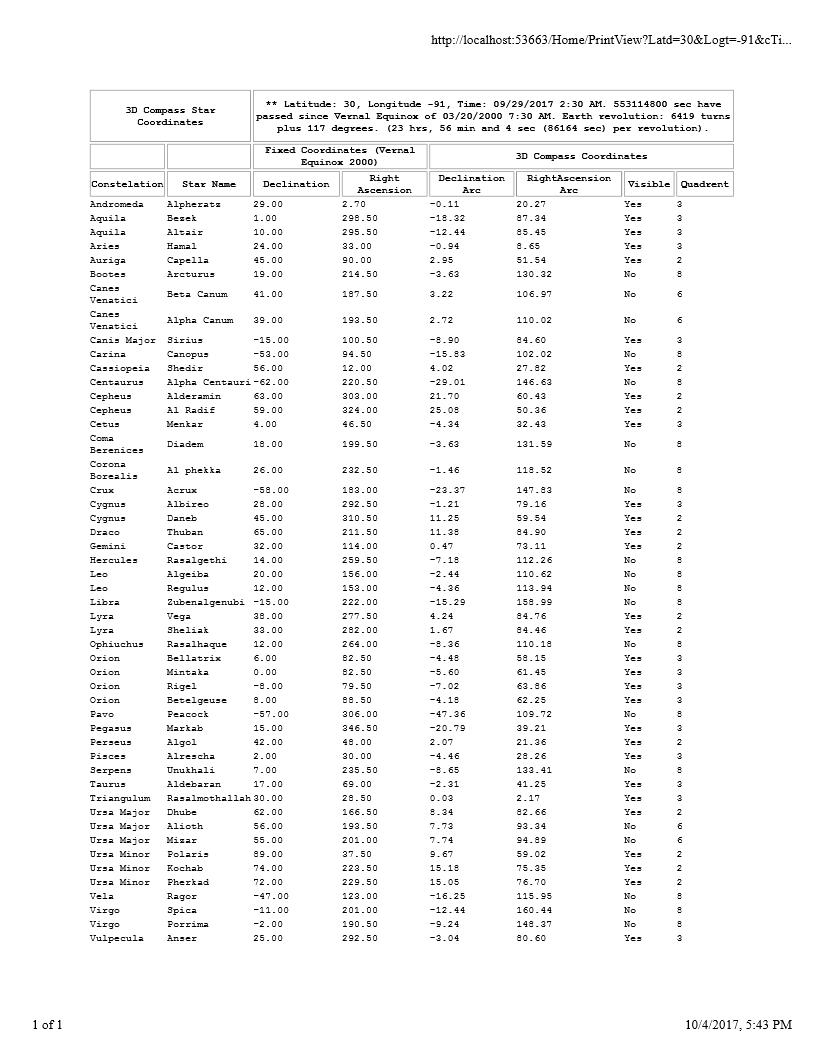Note

Notes

# Workshop

Exercise 1
Calculate number of revolutions earth has made since Vernal Equinox of Mar 20, 2000 7:30 AM (GMT) till September, 29, 2:30 AM. (earth takes 23 hours, 56 minutes and 4 second to revolve around its own axis.)
9/20/2017 2:30 AM – 3/20/2000 7:30 = 553622400 seconds.
Earth revolution: 553114800 / 86164 = 6419 20 turns plus 117 degrees.
Exercise 2
Calculate the Arc length  of star Betelgeuse from earth latitude 30o, longitude -91o at the time October, 4, 11:30 PM. (The celestial coordinates of star Betelgeuse are 8o Declination, 88.5o Right Ascension.) The Arc indicates the Right Ascension angle for 3D Compass.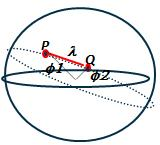Fig 1.30. Great circles
Earth has shifted 117o from Celestial coordinates (see Exercise 1).
Reference longitude = -91 + 117 = 26o, Reference latitude = 30o. Point P (30,26).
Star Declination = 8o, Star Right Ascension 88.5o. Point Q (8, 88.5).
Δφ = cos-1(cos(φ1) x cos(φ2) x cos(λ) + sin (φ1) x sin(φ2))
Δφ = cos-1(cos(30) x cos(8) x cos(88.5-26)) + sin (30) x sin(8))
Δφ = 62.5o

Exercise 3
Draw a point facing north equal distance of arc length from reference location (30,26) to star coordinate (8,88,5).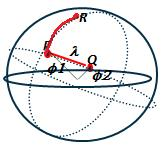Fig 1.31. Great circle angles
Simply add the arc length 62.5o (from exercise 2) to the reference latitude.
Point R = ((30 + 62.5), 26) = 92.5o,26o
Exercise 4
Convert polar coordinates of point P, R and Q into rectangular coordinates
Point P (26,30),
Px = cos(30)x(cos(26) = 0.78, Py=cos(30)xSin(30) = 0.38, Pz = Sin(30) = 0.5.
Point Q (8,88.5),
Qx = cos(8)x(cos(88.5) = 0.026, Qy=cos(8)xSin(88.5) = 0.99, Qz = Sin(8) = 0.14.
Point R (92.5,30),
Rx = cos(8)x(cos(88.5) = -0.04, Ry=cos(8)xSin(88.5) = -0.017, Rz = Sin(8) = 0.99

Exercise 5
Convert to Vector PR (point P and point R) and convert to Vector PQ (Point P and point Q)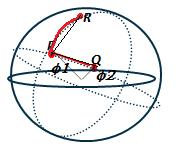Fig 1.31. Vector PR and PQ
X = Rx – Px Y= Ry- Py, Z = Rz - Pz
X = -0.04 – 0.78 = -0.81, Y = -0.017 – 0.38 = -0.4 Z = 0.5
Vector PQ
X = Qx – Px Y= Qy- Py, Z = Qz - Pz
X = 0.026 – 0.78 = -0.75, Y = 0.99 – 0.38 = 0.61 Z = -0.36

Exercise 4
Compute Vector magnitude PR and PQ.
Magnitude of Vector PR = x2+y2+z2=-0.812+-0.42+0.52=1.03
Magnitude of Vector PQ = x2+y2+z2=-0.752+0.612+-0.362=1.03
Exercise 5
Compute dot product of the Vector PR and PQ.

DotProduct = PR●PQ = PRx*PQx+PRy*PQy+PRz*PQz =
PR●PQ = -0.81*-0.75+0.4*0.61+0.5* -0.36=0.19

Exercise 5
Compute angle  between the Vector PR and PQ.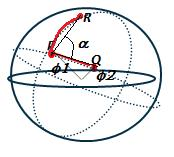Fig 1.32. Angle between vectors
α=cos-1dot productmagnitude A*Magnitude B
α=cos-10.191.03*1.03=80 degree

Note

Notes